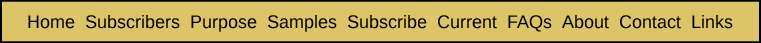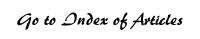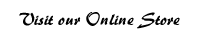An Equality That Simplifies the Calculation of the Probability of a Crossing Regardless of the Number of Occurrences of an ELS Appendix Six from Breakthrough: Encountering the Reality of the Bible Codes As we saw in Appendix Five, trying to calculate the probability that a given ELS will cross a stated section of text can expand into the task of generating a large table of numbers. This chore can be avoided, however, by the use of the following formula.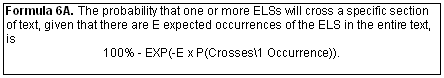That this one simple calculation can provide the same result as that produced from creating an extensive table like the one shown at the end of Appendix Five is quite surprising. It is also very helpful. While that table results in a probability of 16.0%, the above formula produces 16.0543%: 100% – EXP(-3.5 x 5%) = 100% – EXP(-0.175) = 16.0543%. Why the difference? Let's look again at the table at the end of Appendix Five: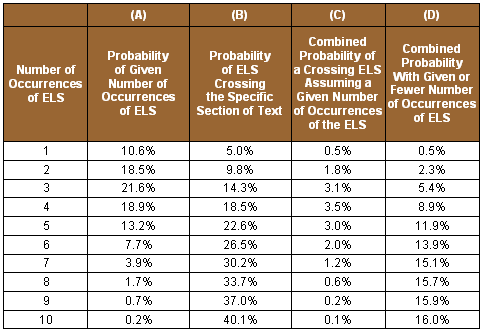The above table only includes situations where the number of occurrences of the ELS in the entire text are 10 or less. In reality, there is some chance that the given ELS could occur 11 or 12 or 13 or more times. The probability of 11 occurrences is 0.073%, of 12 occurrences is 0.0213%, and of 13 is 0.0057%. By including those situations, the final probability rises from the 16.0% in the table to the 16.0543% indicated by the formula. This can be seen below when we extend the above table up to 16 occurrences of the ELS and show more decimal places.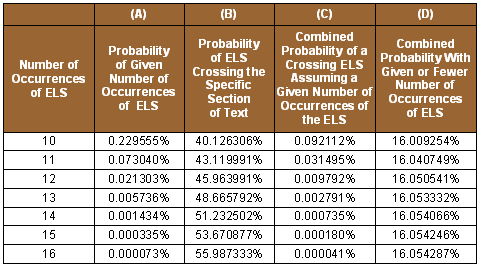In the remainder of this Appendix, we will provide a rigorous mathematical proof of Formula 6A. Since we know of no simple way to present this proof, it is provided with the comment that understanding the rest of this Appendix generally will require a college level of knowledge of mathematics. In terms of the above example, what we want to derive is the simple equation shown in Formula 6A for the last number in Column (D), 16.0543%. We will give this probability the symbol of capital p, or P. How is that number derived in terms of the table? It is the sum of the probabilities in Column (C). Each of those probabilities is the product of the corresponding values in Columns (A) and (B). In other words, one formula for the desired probability (P) at the bottom of Column (D) is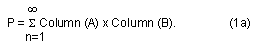Now Column (A), for any given value of n, is the probability that the ELS will occur exactly n times in the entire text. We will denote that probability as p(n). Column (B), for any given value of n, is the probability that the ELS will cross the specific section of text. We will denote that probability as c(n). This turns formula (1a) into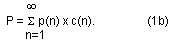In Appendix 5, we noted that c(n) is given by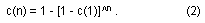For simplicity we will denote c(1) as c. Using the above notation and formulae, we obtainBy breaking (3) into separate terms, we have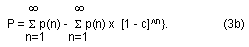Now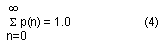since it is the sum of the probabilities of all possible events. Clearly,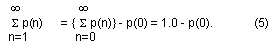What is next needed is a formula for p(n). In Appendix Five, it was noted that p(n) is a Poisson probability. In Appendix Two, we noted that the formula for the Poisson probability of any given number (n) of occurrences of an event, given that its expected number of occurrences is E, is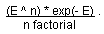Given this,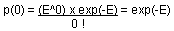Substituting this for p(0) in formula (5) yields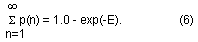If we substitute the formula for the Poisson probability for p(n) and also use formula (6) and restate formula (3b), we get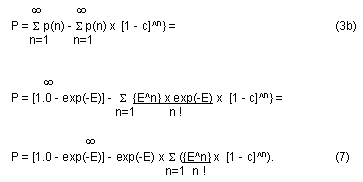Now the power series formula for exp(a) is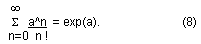For the case where the summation starts with n=1, we have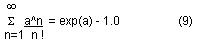since a^0/0! equals 1.0. In formula (7), a = E(1–c), therefore, the summation in formula (7) becomes exp(E[1–c]). Making this substitution in formula (7), we obtainSince exp(-E) x exp(E) = 1, (10) becomesThis is Formula 6A, so we have completed the proof.

 Enjoy finding your own Bible codes. Bible code search software is available in our online store. Subscribe Free! By signing up to be a member of The Isaac Newton Bible Research Society, you will have access to more than fifteen years of research by our team of Bible code researchers. Sign up to be a member today.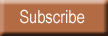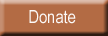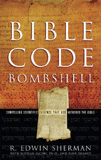Bombshell examines two massive, recently discovered clusters of codes in the Hebrew Old Testament. To read more about Bombshell, click here, or click below to order from Amazon today!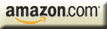| HOME | SUBSCRIBERS ONLY | OUR PURPOSE | FREE SAMPLES | CURRENT | | FAQs | ABOUT BIBLE CODES | SUBSCRIBE | CONTACT | Copyright © 2016 BibleCodeDigest.com